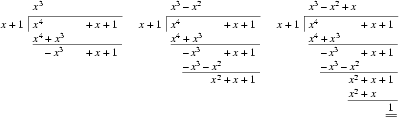odd doctor 已更新

# Polynomial Remains

Time Limit
1s
Memory Limit
65536KB
Judge Program
Standard
Ratio(Solve/Submit)
100.00%(1/1)
Description:Given the polynomial

a(x) = an xn + ... + a1 x + a0,

compute the remainder r(x) when a(x) is divided by xk+1.

Input:

The input consists of a number of cases. The first line of each case specifies the two integers n and k (0 <= n, k <= 10000). The next n+1 integers give the coefficients of a(x), starting from a0 and ending with an. The input is terminated if n = k = -1.

Output:

For each case, output the coefficients of the remainder on one line, starting from the constant coefficient r0. If the remainder is 0, print only the constant coefficient. Otherwise, print only the first d+1 coefficients for a remainder of degree d. Separate the coefficients by a single space.

You may assume that the coefficients of the remainder can be represented by 32-bit integers.

Sample Input:
5 2
6 3 3 2 0 1
5 2
0 0 3 2 0 1
4 1
1 4 1 1 1
6 3
2 3 -3 4 1 0 1
1 0
5 1
0 0
7
3 5
1 2 3 4
-1 -1

Sample Output:
3 2
-3 -1
-2
-1 2 -3
0
0
1 2 3 4


Submit